Ch 2. Derivatives I Multimedia Engineering Math DerivativeFormulas TrigonometricFunctions Differentiability ChainRule ImplicitDifferentiation
 Chapter 1. Limits 2. Derivatives I 3. Derivatives II 4. Mean Value 5. Curve Sketching 6. Integrals 7. Inverse Functions 8. Integration Tech. 9. Integrate App. 10. Parametric Eqs. 11. Polar Coord. 12. Series Appendix Basic Math Units Search eBooks Dynamics Fluids Math Mechanics Statics Thermodynamics Author(s): Hengzhong Wen Chean Chin Ngo Meirong Huang Kurt Gramoll ©Kurt GramollMATHEMATICS - THEORY

The main topic of this section is derivative formulas for trigonometric functions.

Trigonometric limits at Specific Angle

In order to compute the derivatives of trigonometric function, some of the trigonometric limits need to be mentioned first.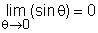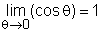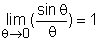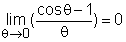Derivatives of Trigonometric Functions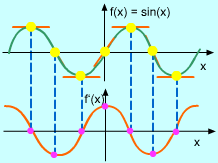sin'x = cos x

The derivatives of trigonometric functions can be proven by use of the definition of derivative and the trigonometric limits.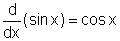(1)

Proof

If f(x) = sinx, then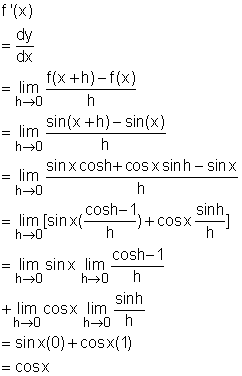Now that d(sinx)/dx equals cosx has been proven, what does d(sinx)/dx look like in graph? Is it the same as cosx?

The value of the derivative at any value of x can be estimated by drawing the tangent at the point (x, f(x)) and estimate its slope. For example, at the point of x equals 0, draw the tangent at this point and estimate its slope to be around 1, so d(sinx)/dx = 1. Now plot the point (0, 1) on the graph of df/dx or f '. By repeating this procedure at different points, the graph of f ' can be drawn and will resemble a cosine curve. The correction of the graph is confirmed by formula (1).

Using the definition of derivative and the trigonometric limits, the formula

d(cosx)/dx = -sinx                                         (2)

can be proved.

According to the formula (1) and (2), the derivative of tangent function can be found.

d(tanx)dx = sec2 x

Proof

If f(x) = tanx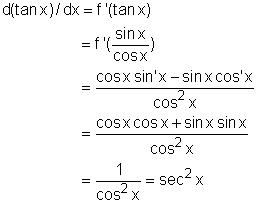The derivatives of the csc, sec, and cot are listed below:

d(secx)/dx = secx tanx

d(cscx)/dx = -cscx cotx

d(cotx)/dx = -csc2x

The follow table is a list of most commonly used derivatives of the trigonometric functions.

 Number Derivatives of the trigonometric functions 1 d(sinx)/dx = cosx 2 d(cosx)/dx = -sinx 3 d(tanx)/dx = sec2x 4 d(secx)/dx = secx tanx 5 d(cscx)/dx = -cscx cotx 6 d(cotx)/dx = -csc2x